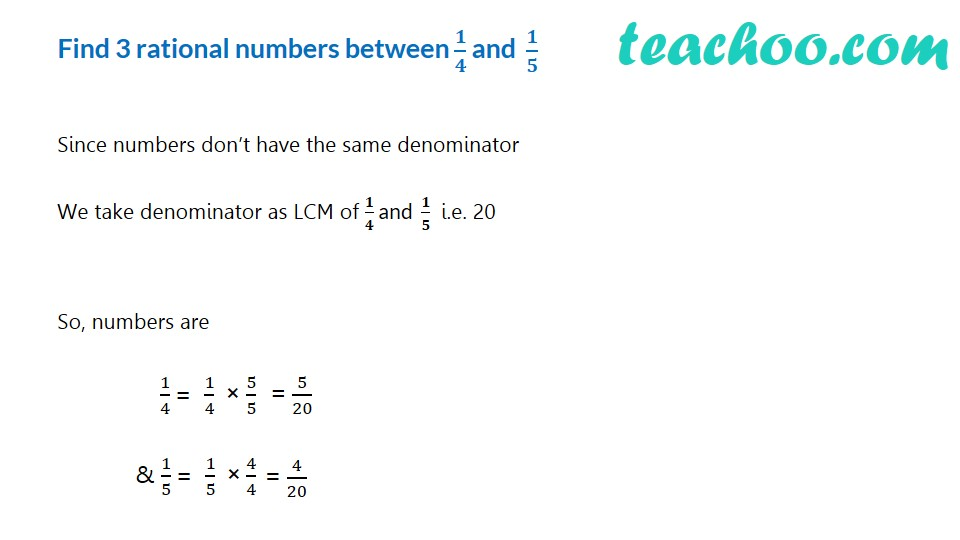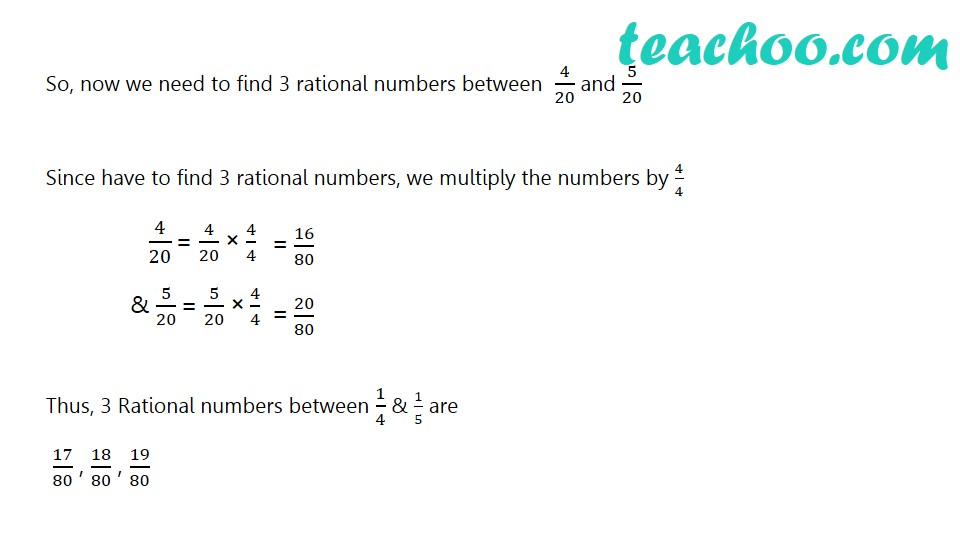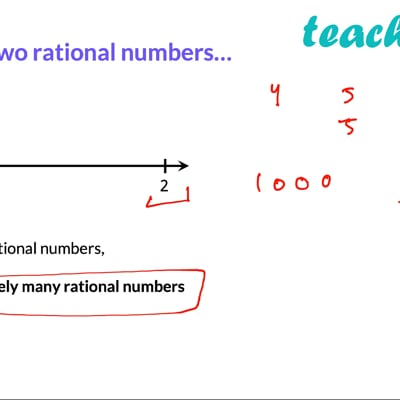Finding rational number between two numbers

Chapter 1 Class 9 Number Systems
Concept wiseThis video is only available for Teachoo black users

Maths Crash Course - Live lectures + all videos + Real time Doubt solving!

### Transcript

Find 3 rational numbers between 𝟏/𝟒 and 𝟏/𝟓 Since numbers don’t have the same denominator We take denominator as LCM of 𝟏/𝟒 and 𝟏/𝟓 i.e. 20 So, numbers are 1/4 = 1/4 × 5/5 & 1/5 = 1/5 × 4/4 So, now we need to find 3 rational numbers between 4/20 and 5/20 Since have to find 3 rational numbers, we multiply the numbers by 4/4 4/20 = 4/20 × 4/4 & 5/20 = 5/20 × 4/4 Thus, 3 Rational numbers between 1/4 & 1/5 are 17/80 , 18/80 , 19/80